# Electrolysis: solved equations

## Introduction

In an Electrolysis application:

• from the electric point of view: the equations used are those of the Electric Conduction application (see the following block)
• from the magnetic point of view: the computation of the magnetic field created by the distribution of the current is carried out by means of the analytical Biot – Savart formula (or semi-analytical).

## Two types of computation

From the electrical point of view, two types of computation can be carried out:

• incomplete computation: (without taking into account the current density inside the metallic structure)

it is a computation of the Electric Conduction type:

• computation of the electric potential in the electrolyte (VEL).
• “complete” computation (taking into account the current density inside the metallic structure)

it comprises a sequence of two computations of the Electric Conduction type:

• phase 1 : computation of the electric potential in the electrolyte (VEL)

⇒ computation of the normal component of the current density on the electrochemical interface

• phase 2 : computation of the electric potential inside the metallic structure (V) while imposing the current densities previously computed on the electrochemical interface

## Solved equation

The second order equation solved by the finite elements method in Flux in case of an Electrolysis application is the following: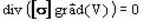where:

• [σ] is the tensor of the conductivity of the medium (in S)
• V is the electric potential (in V)

## State variable

The state variables are the following electric potentials:

• VEL : electric potential in the electrolyte (electric potential of the incomplete computation or of phase 1 of the complete computation)

• V: electric potential in the metal parts (electric potential of phase 2 of the complete computation)

The uniqueness condition of the scalar field of the electric potential V requires that the value of this potential be assigned to at least one point of the computation domain.

The electric potential (VEL) in the electrolyte region is generally called UEP (Underwater Electric Potential)

## Analogy with a thermal problem

For an incomplete computation, the equations are the same as for the thermal applications. The correspondence between the electric and thermal quantities is presented in the table below.

Electric Conduction application

Electric potential

V

Temperature

T

Electric conductivity

σ

Thermal conductivity

k

Current density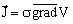Heat flux density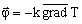Cathodic potential

Vc

Ambiant temperature

Ta

Condition with polarization law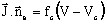Condition with convection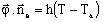Coefficient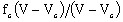Convection coefficient

h